# Naive matrix multiplication

## Definition

Naive matrix multiplication refers to the naive algorithm for executing matrix multiplication: we calculate each entry as the sum of products.

Explicitly, suppose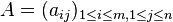is a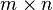matrix and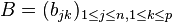is a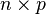matrix, and denote by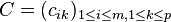the product of the matrices. We then have the following formula: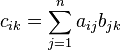In other words, each entry of the product is computed as a sum ofpairwise products.

## Arithmetic complexity

### Complexity for the serial algorithm without parallelization

• The total number of multiplications is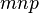: there aremultiplications required to calculate each entry, and there are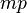entries to be calculated.
• Under the assumption that our algorithm allows only for the addition of numbers two at a time, the total number of additions required is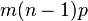: To compute each entry, we need to addproducts. This requiresadditions (we add the first two, then the third to the sum, and so on). There areentries in total, so we get a total addition count of.
Case Arithmetic description Number of multiplications needed Number of additions needed
Both matrices are square matrices of the same size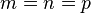; we will usefor these equal numbers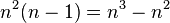The first matrix is a row matrix and the second matrix is a column matrix, so the product is amatrix and the product is a dot product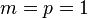,can be arbitraryThe first matrix is a column matrix, the second matrix is a row matrix, so the product is a Hadamard product (outer product)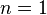; the values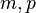can be arbitrary0
The product matrix is a square matrix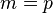(we will denote this equal value as);can be arbitrary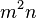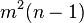### Complexity allowing for parallelization

The arithmetic complexity allowing parallelization (ignoring communication complexity issues entirely) is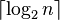. The reasoning is as follows:

• The computations of all the matrix entries can be done in parallel, because the computations for the entries do not depend on each other.
• For computing a given entry, all the computations of the products being added can be done in parallel. Combined with the preceding observation, we obtain that all themultiplications can be performed in parallel.
• The only thing that cannot be completely parallelized is the addition step. However, this too can be partly parallelized. We can divide the list of terms to be added into two halves, sum them up, and combine. By iterating this process of dividing in halves and adding, we can encode the parallelized addition using a binary tree. The arithmetic time complexity is then given by the depth of the tree, which is.

In practice, no matrix multiplication algorithm would be that fast, because of communication complexity issues: it is unrealistic to expect that all the parallel processors will be able to read and write the main data at zero cost.

## Variants given special structure to the matrices

If we are given prior guarantees that the matrix has a particular structure, and preferably an encoding of the matrix that exploits the structure, then even the "naive" matrix multiplication algorithm may be improvable. In particular, if we know a specific subset of the entries is guaranteed to be zero, we can save on the effort of computing the summands that are products of those terms.

Type of matrices Number of multiplications under naive matrix multiplication, not accounting for structure Number of additions under naive matrix multiplication, not accounting for structure Number of multiplications under naive matrix multiplication, accounting for structure Number of additions under naive matrix multiplication, accounting for structure Big-Oh saving?
Both arediagonal matrices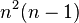0 Yesisdiagonal,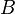is arbitrary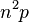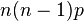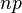0 Yes
Both areupper triangular matrices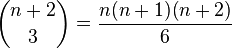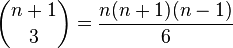No